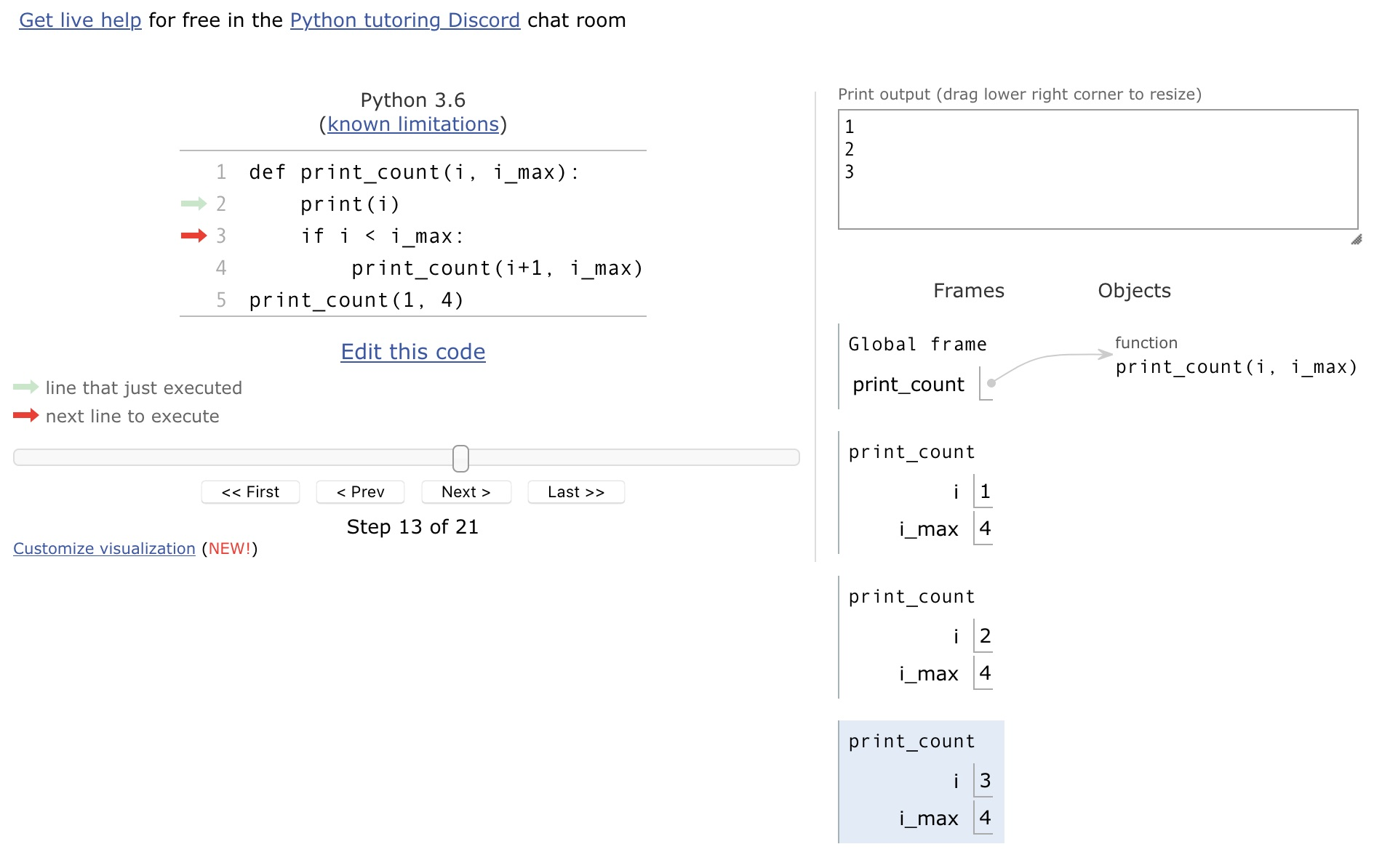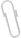# Intro toComputerScience

Fall 2020
##### site-->

# recursion

Our last topic of the course is recursion - a powerful idea that you'll see more of if you continue on and take an algorithms course.

This material covers some of the same material as in Zelle's chapter 12.

In addition to the basic idea, I'll show several examples of what these ideas can be used for, with python code that uses only the syntax and data structures that we've covered.

## the main idea

Definition: recursion.
See "recursion".


Whenever a problem has smaller pieces that look like the original problem, it's possible to use an approach in which the answer is defined in terms of itself. At first this seems almost like magic. But as long the code has a stopping point built-in, it works.

Consider this code.

def print_count(i, i_max):
print(i)
if i < i_max:
print_count(i+1, i_max)
print_count(1,4)


This prints the numbers from 1 to 4. It's sort of a loop ... but without the loop. The thing that makes this recursive is that the print_count function calls itself from within the body of the function.

Here's what it looks like in pythontutor.com, part way through its execution.Notice on the right that there are multiple instances of the function which are in memory and running at the same time, each with a different value for its local variable i.

This is one of the problems with recursion, at least the way it's implemented in python - if we tried to count to a million this way, python would try to store a million copies of the running function, and we'd get a memory error. Some languages support "tail recursion", which can be a way to avoid this memory problem.

## lists and trees

Two data structures which have the "self-similar" property that lets them work well with recursion are lists and trees.

A list like [1,2,3,4] can be though of as made up of a leading 1, then the rest of the list [2,3,4] ... which is also a list. We can use that to write for example this function to find the length of a list.

def length(a_list):
if a_list == []:          # Empty list? Length is 0.
return 0
else:                     # Not empty?
all_but_first = a_list[1:]
return 1 + length(all_but_first)  # 1+length(rest)


This sort of idea becomes even more powerful when we're working with graphs or trees - data structures that have branches that make them more complicated to loop over. Since each sub-tree is also a tree, the same trick can be used.

Here's an example of a small binary tree (each "node" has two things under it) implemented with python objects, and a few recursive functions : binarytree.py .

## fractals

Another use of recursion is in drawing self-similar images - fractals.

Here's a short program to draw the Koch Curve, using a turtle graphics library which is built into python. (The turtle moves on the screen, drawing as it goes, like an etch-a-sketch).And here are lots more recursive fractal examples, generated in the "contextfree" language.

It isn't python, but is a nice collection of images defined with recursion. Something along these lines could be accomplished with Zelle's graphic library as a possible final project.

## solving puzzles with recursive search

Many games and puzzles can be thought of having a tree of possible moves, and searching that tree for a solution can be approached with recursion.

Here are three examples , in increasing amounts of code complexity.

Once the objects and methods are set up for a particular situation, for example representing the triangular peg board as an object with methods for printing it, finding the available moves, making a move on the board and so on, the code to actually search recursively for the solution is fairly compact and understandable.

Here is the code that searches for the solution to the peg solitaire puzzle.

def solve(board):
""" Search for a solution. Return True if found,
leaving board in a solved state. """
# ... using a recursive backtracking search.
board.n_positions += 1                 # number of positions examined.
if board.is_solved():
return True
else:
for move in board.moves:
if board.can_move(move):
board.do_move(move)
if solve(board):           # recursively look at the next position
return True
board.undo_move(move)
return False


All my recursion code exapmles are in ../code/recursion.

https://cs.bennington.college /courses /fall2020 /introcs /notes /9b_recursion
## attachmentslast modified sizeprint_count_screenshot.jpg Mon Oct 05 2020 05:55 pm 171K